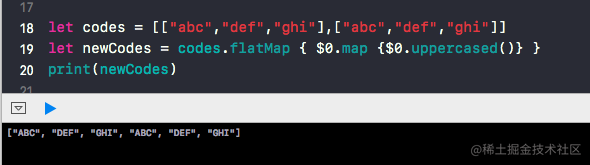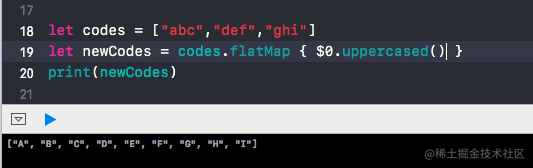# Swift中的高阶函数: Filter, Map, Reduce, flatmap, compactMap(译)

``````// 将函数当做参数传递给另一个函数

func addation(num1: Double, num2: Double) -> Double {
return num1 + num2
}

func multiply(num1: Double, num2: Double) -> Double {
return num1 * num2
}

func doMathOperation(operation: (_ x: Double, _ y: Double) -> Double, num1: Double, num2: Double) -> Double {
return operation(num1, num2)
}

// print 12
doMathOperation(operation: addation(num1:num2:), num1: 10, num2: 2)
//print 20
doMathOperation(operation: multiply(num1:num2:), num1: 10, num2: 2)

`addation(num1:num2:)``multiply(num1:num2:)`都是`(Double,Double)->Double`的函数。`addation(num1:num2:)`接受两个 Double 的值，并返回它们的和；`multiply(num1:num2:)`接受两个 Double 的值，并返回它们的乘积。`doMathOperation(operation:num1:num2:)`则是一个接受三个参数的高阶函数，它接受两个 Double 的参数和一个`(Double,Double)->Double` 类型的函数。看一下该函数的调用，你应该就理解了高阶函数的工作原理。

``````// 函数返回值为另一个函数
func doArithmeticOperation(isMultiply: Bool) -> (Double, Double) -> Double {
func addation(num1: Double, num2: Double) -> Double {
return num1 + num2
}

func multiply(num1: Double, num2: Double) -> Double {
return num1 * num2
}

return isMultiply ? multiply : addation
}

let operationToPerform1 = doArithmeticOperation(isMultiply: true)
let operationToPerform2 = doArithmeticOperation(isMultiply: false)

operationToPerform1(10, 2) //20
operationToPerform2(10, 2) //12

### Map

map 函数的作用就是对集合进行一个循环，循环内部再对每个元素做同一个操作。它返回一个包含映射后元素的数组。

#### Map on Array：

``````let arrayOfInt = [2,3,4,5,4,7,2]

``````var newArr: [Int] = []
for value in arrayOfInt { newArr.append(value*10) }
print(newArr) // prints [20, 30, 40, 50, 40, 70, 20]

``````let newArrUsingMap = arrayOfInt.map { \$0 * 10 }

map 的工作原理：map 函数接受一个闭包作为参数，在迭代集合时调用该闭包。这个闭包映射集合中的元素，并将结果返回。map 函数再将结果放在数组中返回。

#### Map on Dictionary：

``````let bookAmount = [“harrypotter”:100.0, “junglebook”:100.0]

``````let bookAmount = ["harrypotter": 100.0, "junglebook": 100.0]
let returnFormatMap = bookAmount.map { (key, value) in
return key.capitalized
}
print(returnFormatMap)
//["Junglebook", "Harrypotter"]

`注意：map 函数的返回值类型总是一个泛型数组。你可以返回包含任意类型的数组。`

#### Map on set:

``````let lengthInMeters: Set = [4.0, 6.2, 8.9]
let lengthInFeet = lengthInMeters.map { \$0 * 3.2808 }
print(lengthInFeet)
//[20.340960000000003, 13.1232, 29.199120000000004]

#### 如果你想在 map 的时候获取 index 应该怎么做？

``````let numbers = [1, 2, 4, 5]
let indexAndNum = numbers.enumerated().map { (index,element) in
return "\(index):\(element)"
}
print(indexAndNum) // [“0:1”, “1:2”, “2:4”, “3:5”]

### Filter

`filter` 会遍历集合，返回一个包含符合条件元素的集合。

#### Filter on array

``````let arrayOfIntegers = [1, 2, 3, 4, 5, 6, 7, 8, 9]

var newArray = [Int]()

for integer in arrayOfIntegers {
if integer % 2 == 0 {
newArray.append(integer)
}
}

print(newArray) //[2, 4, 6, 8]

``````var newArray = arrayOfIntegers.filter { (value) -> Bool in
return value % 2 == 0
}

filter 闭包也可以被简化，就像 map：

#### Filter on dictionary

``````let bookAmount = ["harrypotter":100.0, "junglebook":1000.0]

filter 函数会调用一个名字为 isIncluded 的闭包，该闭包接受一个键值对作为参数，并返回一个布尔值。最终，基于返回的布尔值，filter 函数将决定是否将键值对添加到数组中。

`<重要> 原文中作者写道：对字典调用 Filter 函数，将返回一个包含元组类型的数组。但译者在 playground 中发现 返回值实际为字典类型的数组。`

``````let bookAmount = ["harrypotter": 100.0, "junglebook": 1000.0]
let results = bookAmount.filter { (key, value) -> Bool in
return value > 100
}

print(results) //["junglebook": 1000.0]

``````//\$0 为 key \$1 为 value
let results = bookAmount.filter { \$1 > 100 }

#### Filter on set

``````let lengthInMeters: Set = [1, 2, 3, 4, 5, 6, 7, 8, 9]
let lengthInFeet = lengthInMeters.filter { \$0 > 5 }
print(lengthInFeet) //[9, 8, 7, 6]

### Reduce

reduce ：联合集合中所有的值，并返回一个新值。

Apple 的官方文档如下：

``````func reduce<Result>(_ initialResult: Result, _ nextPartialResult: (Result, Element) throws -> Result) rethrows -> Result

reduce 函数接受两个参数：

• 第一个为初始值，它用来存储初始值和每次迭代中的返回值。
• 另一个参数是一个闭包，闭包包含两个参数：初始值或者当前操作的结果、集合中的下一个 item 。

#### Reduce on arrays

``````let numbers = [1, 2, 3, 4]
let numberSum = numbers.reduce(0) { (x, y)  in
return x + y
}
print(numberSum) // 10

reduce 函数会迭代4次。

``````1.初始值为0，x为0，y为1 -> 返回 x + y 。所以初始值或者结果变为 1。
2.初始值或者结果变为 1，x为1，y为2 -> 返回 x + y 。所以初始值或者结果变为 3。
3.初始值或者结果变为 3，x为3，y为3 -> 返回 x + y 。所以初始值或者结果变为 6。
4.初始值或者结果变为 6，x为6，y为4 -> 返回 x + y 。所以初始值或者结果变为 10。

reduce 函数可以简化为：

``````let reducedNumberSum = numbers.reduce(0) { \$0 + \$1 }
print(reducedNumberSum) // prints 10

``````let reducedNumberSum = numbers.reduce(0,+) // returns 10

``````let reducedNumberSum = numbers.reduce(0) { \$0 * \$1 }
// reducedNumberSum is 0...

``````let reducedNumberSum = numbers.reduce(0,*)

reduce 也可以通过 + 操作符来合并字符串。

``````let codes = ["abc","def","ghi"]
let text = codes.reduce("") { \$0 + \$1} //the result is "abcdefghi"
or
let text = codes.reduce("",+) //the result is "abcdefghi"

#### Reduce on dictionary

``````let bookAmount = ["harrypotter":100.0, "junglebook":1000.0]

let reduce1 = bookAmount.reduce(10) { (result, tuple) in
return result + tuple.value
}
print(reduce1) //1110.0

let reduce2 = bookAmount.reduce("book are ") { (result, tuple) in
return result + tuple.key + " "
}
print(reduce2) //book are junglebook harrypotter

``````1.一个应该被 reduce 的初始值或结果
2.一个当前键值对的元组

reduce2 可以被简化为：

``````let reducedBookNamesOnDict = bookAmount.reduce("Books are ") { \$0 + \$1.key + " " } //or \$0 + \$1.0 + " "

#### Reduce on set

Set 中的 reduce 使用和数组中的一致。

``````let lengthInMeters: Set = [4.0, 6.2, 8.9]
let reducedSet = lengthInMeters.reduce(0) { \$0 + \$1 }
print(reducedSet) //19.1

### Flatmap

Flatmap 用来铺平 collections 中的 collection 。在铺平 collection 之前，我们对每一个元素进行 map 操作。 `Apple docs 解释: 返回一个对序列的每个元素进行形变的串级结果( Returns an array containing the concatenated results of calling the given transformation with each element of this sequence.)`

``````解读 : map + (Flat the collection)`图1 对 flatmap 进行代码说明``图2`

1. 对所有的字符串执行 `upperCased()` 函数，这类似于:
``````[“abc”,”def”,”ghi”].map { \$0.uppercased() }

``````output: [“ABC”, “DEF”, “GHI”]

1. 将 collections 铺平为一个 collection。
``````output: ["A", "B", "C", "D", "E", "F", "G", "H", "I"]

`注意：在 Swift3 中，flatMap 还可以自动过滤 nil 值。但是现在已经废弃该功能。现在用 compactMap 来实现这一功能，稍后在文章中我们会讲到。`

#### Flatmap on array

``````let arrs = [[1,2,3], [4, 5, 6]]
let flat1 = arrs.flatMap { return \$0 }
print(flat1) //[1, 2, 3, 4, 5, 6]

#### Flatmap on array of dictionaries

``````let arrs = [["key1": 0, "key2": 1], ["key3": 3, "key4": 4]]
let flat1 = arrs.flatMap { return \$0 }
print(flat1)
//[(key: "key2", value: 1), (key: "key1", value: 0), (key: "key3", value: 3), (key: "key4", value: 4)]

var dict = [String: Int]()

flat1.forEach { (key, value) in
dict[key] = value
}

print(dict)
//["key4": 4, "key2": 1, "key1": 0, "key3": 3]

#### Flatmap by filtering or mapping

``````let collections = [[5, 2, 7], [4, 8], [9, 1, 3]]
let onlyEven = collections.flatMap { (intArray) in
intArray.filter({ \$0 % 2 == 0})
}
print(onlyEven) //[2, 4, 8]

``````let onlyEven = collections.flatMap { \$0.filter { \$0 % 2 == 0 } }

### 链式 : (map + filter + reduce)

``````let arrayOfArrays = [[1, 2, 3, 4], [5, 6, 7, 8, 4]]
let sumOfSquareOfEvenNums = arrayOfArrays.flatMap{\$0}.filter{\$0 % 2 == 0}.map{\$0 * \$0}.reduce {0, +}
print(sumOfSquareOfEvenNums) // 136

//这样可以运行
let SquareOfEvenNums = arrayOfArrays.flatMap{\$0}.filter{\$0 % 2 == 0}.map{\$0 * \$0}
let sum = SquareOfEvenNums.reduce(0 , +) // 136

### CompactMap

``````let arr = [1, nil, 3, 4, nil]
let result = arr.compactMap{ \$0 }
print(result) //[1, 3, 4]

``````let nums: Set = [1, 2, nil]
let r1 = nums.compactMap { \$0 }
print(r1) //[2, 1]

``````let dict = ["key1": nil, "key2": 20]
let result = dict.compactMap{ \$0 }
print(result) //[(key: "key1", value: nil), (key: "key2", value: Optional(20))]

let dict = ["key1": nil, "key2": 20]
let result = dict.compactMapValues{ \$0 }
print(result)

#### Tip

``````let arr = [1, nil, 3, 4, nil]
let result = arr.map { \$0 }
print(result) //[Optional(1), nil, Optional(3), Optional(4), nil]

### 总结

• 它可以提高你的 Swift 技能
• 代码可读性更高
• 更加函数式

iOS

iOS